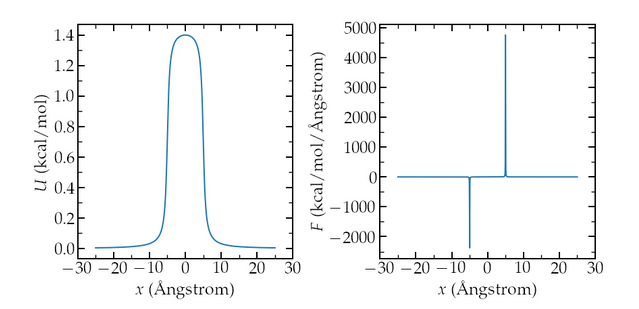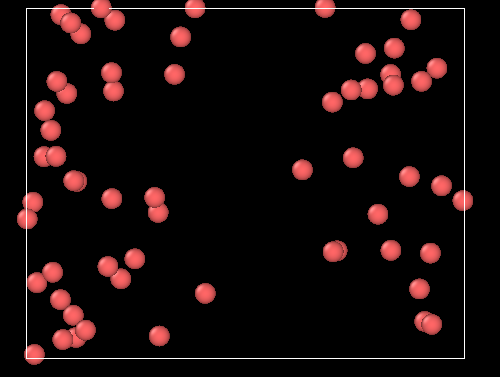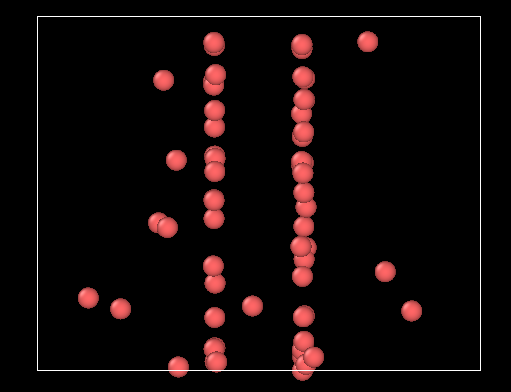# Pure Monte Carlo simulation with fix addforce: unexpected behavior? [solved]

Dear LAMMPS users/developpers,

I am currently writing a small tutorial to explain the difference between molecular dynamics (MD) and Monte Carlo (MC) simulations. The system is a simple box with Lennard Jones atoms. The center of the box is made energetically unfavorable for the atoms thanks to the addforce command which add an extra potential to the atoms. The extra potential U (and force F) looks like that :If I run the MD simulation (NVT ensemble), I’ve got the expected behavior very quickly, ie the atoms are (in average) excluded from the center of the box:If I run a pure MC simulation, however, using the fix GCMC as follow :

fix mymc all gcmc 1 0 5 1 29494 119.8 -5 1 full_energy

I’ve got the following weird behavior, where the atoms accumulate where the absolute value of the force is maximale :I’ve tried:

1. different parameters for the added force → weird behavior still occurring
2. removing the fix_modify energy yes from the addforce or/and the full_energy from the gcmc → in that case atoms behave as if no extra force was added, as expected from the doc
3. play with the displace value of the gcmc → weird behavior still occurring
4. perform hybrid MC/MD run → weird behavior still occurring
5. use different number of MC move → weird behavior still occurring
6. remove the pair_modify shift yes and change the cut-off of the LJ to 1 nm → weird behavior still occurring

Here is a minimal script to do either the MD or the MC simulation, depending on what is commented:
input.lammps (898 Bytes)

Am I missing something obvious here? Or is this a bug?

LAMMPS version : lammps-2Jun2022

Best,
Simon

Not obvious, but it is documented. For force as a variable, you have to define the corresponding energy as a variable, or else it is set to zero.

`variable F atom \${U0}/((x-\${x0})^2/\${dlt}^2+1)/\${dlt}-\${U0}/((x+\${x0})^2/\${dlt}^2+1)/\${dlt}`
`variable E atom (\${U0}/((x-\${x0})/\${dlt}^2+1)/\${dlt}-\${U0}/((x+\${x0})/\${dlt}^2+1)/\${dlt})`
`fix myadf all addforce v_F 0.0 0.0 energy v_E`

Thank you very much for your answer, I missed this one in the doc.

For completeness, here is a fully working input script that combine addforce and monte carlo move :
input.lammps (841 Bytes)

1 Like